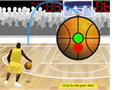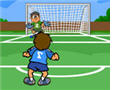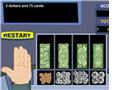#One-Digit Addition Game

Practice adding one-digit whole numbers when playing this fun basketball math gameAdding One-Digit Numbers

In this interactive soccer math game, students will add whole numbers smaller than ten.One-Digit Addition Game
One-Digit Plus Two-Digit NumbersAdding Two-Digit Numbers

In this game students will add two-digit whole numbers.Multi-Digit Addition Game

In this basketball math game, kids will show off their skills by adding different decimals.Adding Decimals Game

Add different decimals correctly to earn points and a chance to kick the ball for additional bonus points.Adding Money Game

In this interactive game about adding money, students will use a virtual cash register to answer each problem.They will have to click on the bills and coins provided in the cash drawer to indicate the correct amount. Some answers might have multiple correct choices.Math Racing - Adding Integers Game

Middle school students will have a lot of fun practicing their skills when playing this fun math racing game about adding integers.